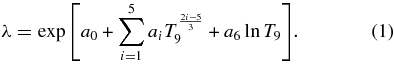# REACLIB Help

Main Page > Thermonuclear Reaction Rate Fitting Suggestions

## Thermonuclear Reaction Rate Fitting Suggestions

Details about the JINA REACLIB Database can be found in Cyburt et al., ApJS 189 (2010) 240.

New rates are fit to the standard seven parameter REACLIB form given in Eq. 1.This format is capable of handling all reaction types. Multiple sets of Eq. 1 with differing parameters, a0 through a6, can be summed in order to properly fit rates with numerous resonant and non-resonant contributions. Non-physical behavior of the reaction rates outside of the fitted temperature range is avoided by enforcing physical constraints on the parameters. The enforcement of physical constraints on the fit parameters is an improvement brought to the REACLIB database with the V1.0 update. Unique rules exist for assigning values to the rate parameters a0-a6 in the cases of charge-induced non-resonant, neutron-induced non-resonant, and narrow resonant rate contributions. In practice these rules should serve more as guidelines so that actual parameter values used are within proximity to those theoretically assigned. For positive Q-value reactions these are summarized in the table.

Shown are the Fitting Rules for Various Types of Reaction Rates, Including Non-resonant (NR) Charge-induced (q-induced) and n-induced Reactions, as well as Narrow Resonant Reactions

Charge-induced Non-Resonant n-induced Non-Resonant Isolated Narrow Resonant
a0 = ln [B(Z1Z2/A)1/3S(0)] a0 = ln [NACΓ(ℓ+3/2)/Γ(3/2) (σv/E)E=0] a0 = ln[DA–3/2ωγ]
a1 = 0 a1 = 0 a1 = –11.6045Er
a2 = -4.2486(Z12Z22A)1/3 a2 = 0 a2 = 0
a3 = float a3 = float a3 = 0
a4 = float a4 = float a4 = 0
a5 = float a5 = float a5 = 0
a6 = –2/3 a6 = ℓ a6 = –3/2
Notes. These rules enforce the proper low-temperature analytics. All parameters with numerical values can be fixed, and those specified as "float" can be varied to accommodate the rate changes at higher temperature.

In the table, NA is Avogadro's number, σ is the cross section in cm2, v is the center of mass relative speed in cm/s, E is the center of mass relative energy in MeV, Z1 and Z2 are the target and reactant charges, A is the reduced mass of the reactants in atomic mass units, S(0) is the astrophysical S-factor in MeV-barn at zero energy, ℓ is the minimum orbital angular momentum value in units of ħ, Γ(z) is the Gamma function, ωγ is the narrow resonance strength in MeV, Er is the narrow resonance energy in MeV, B=7.8318×109 cm3 s-1 mole-1 MeV-1 barn-1, C=0.08617 MeV and D=1.5394×1011 cm3 s-1 mole-1 MeV-1. Reaction rates from shell model or statistical model calculations with high level densities should follow the non-resonant prescriptions. If a rate is comprised of both non-resonant and resonant pieces, multiple sets of the 7-parameter fits may be used to describe the rate. It may also be necessary to fit multiple sets to obtain the requisite precision. Proper use of REACLIB form and fitting procedure should yield a reaction rate fit that is within 5% of the data. 5% fit accuracy is adhered to in the database and is considered acceptable by the authors who note that experimental error is rarely better than 10-15% and theoretical errors are often more than 30%. It may occur that a fit to 5% precision is not possible. Such cases are listed on an automatically generated deviations list and an effort is made to constantly improve such cases. Future updates may include adding 2 more terms in each exponential set (i.e. T97/3 and T93 terms), to improve fitting performance and fit precision.
If you have any reaclib/database questions or problems please email us. Send Email
Last updated by stygstra on November 29th 2010 at 14:53